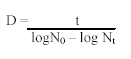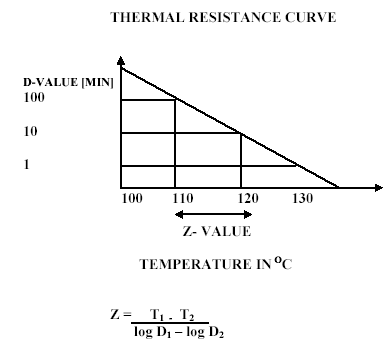## D VALUE, Z VALUE AND F VALUE

D Value, Z Value, F Value & 12-D process is also available as course for better understanding. View Course >

Sterile:- Free from viable micro-organisms.
Sterilization:- Any physical or chemical process which destroys all life forms, with special regard to microorganisms (including bacteria and sporogenous forms), and inactivates viruses.
Therefore the terms “sterile” and “sterilization”, in a strictly biological sense, describe the absence or destruction of all viable micro-organisms. In other words, they are absolute terms: an object or system is either “sterile” or “non-sterile”. The destruction of a microbial population subjected to a sterilization process follows a logarithmic progression. Therefore, only a treatment of infinite duration provides the absolute certainty that the entire microbial population has been destroyed and that the system is sterile. Making the characteristics of the sterilization treatment more drastic (i.e. increasing time and/or temperature) usually entails a decay of the qualities of the product and certainly increases process costs. It is therefore agreed that the product is acceptable as sterile when the probability of finding a non-sterile unit in a sterilized batch entails a risk which is lower than the other risks associated with the use of the product itself. More properly, in the pharmaceutical industry, in order to define a unit as sterile we must be able to certify, on a statistical basis related to the conditions of preparation and sterilization of that specific product and of that specific batch, that less than one unit in a million is exposed to the risk of not being sterile.
The probability of finding a non-sterile unit (PNSU = Probability of Non-Sterile Unit) must therefore be lower than 10-6.
UHT Aseptic Technology (Ultra High Temperature Sterilization):- A sterilization process is defined as a UHT (Ultra High Temperature) process, if the product is heat-treated in a continuous flow at a temperature of not less than 135℃ for a very short time, aseptically packaged in sterile containers, and has undergone minimum chemical, physical and Organoleptic changes in relation to the severity of the heat treatment required for sterilization.
Thermal Death Time (TDT):- Thermal death time is the amount of time that is necessary to kill a specific number of microbes at a specific temperature. This value is obtained by keeping temperature constant and measuring the time necessary to kill the number of cells specified.

##### Decimal reduction time (D-value):- The D-value, which denotes the decimal reduction time, is the time required at a specific temperature and under specified conditions to reduce a microbial population by one decimal. The decimal reduction time is dependent on the temperature, the type of microorganism and the composition of the medium containing the microorganism. Thus, after an organism is reduced by 1 D, only 10% of the original organisms remain. The population number has been reduced by one decimal place in the counting scheme. When referring to D values it is proper to give the temperature as a subscript to the D. For example, a hypothetical organism is reduced by 90% after exposure to temperatures of 300F for 2 minutes, Thus the D-value would be written as D300F = 2 minutes.

It is often more convenient to use the D-value as a measure of rate of microbial inactivation. The D-value is the exposure time required for the number of survivors to change by a factor of 10 or the time required to achieve a decrease of one log cycle in the survivor curve, in other words the temperature or radiation dosed required to reduced the initial population by 90% . The D-value may be estimated graphically see graph or mathematically from the equationNo = bioburden of the chosen bacterium
Nt= surviving population after an exposure time
The D-value and K are specific for each set of microorganisms and each sterilization process. Thus, with data for heat inactivation of microbes the temp is shown D121 ℃. For radiation inactivation, the d-value is stated in the terms absorbed dose (kGy).
D-value is the time required to kill 90% of the spores or vegetative cells of a given microorganism at a specific temperature in a specific medium. D-values can be determined from survivor curves when the log of population is plotted against time, or by the formula:
Dreference temperature = Time/(Loga-Logb)
Where a = the initial population, and b = the survivors after a time interval

##### The 12-D Process:- Canned foods are susceptible to the spores of the organism Clostridium botulinum. This is the organism that causes botulism. These bacterial spores can survive many heat treatment processes. However, in modern food production, canned foods are subjected to a time/temperature process that will reduce the probability of the survival by the most heat-resistant C. botulinum spores by 12 logs or 12-D at 250℉ (the temperature used in the calculation of most commercial 12-D processes is 250℉, and the D-value for this organism at 250℉ is 0.21 minutes). This process is based on the assumption of the number of surviving spores in one can. If we assume that there are 10 surviving spores in one can, then we can calculate the time for a 12-D process to occur by using the following formula:
• F0 = D250(log a – log b), where a = initial population and b = final population.
• So F0 = (0.21min.)(log 101 – log 10-11), we move down 12 log values (1 – (-11)) = 12
• So, F0 = (0.21min.)(1 – (-11)), or 0.21 x 12 = 2.52 minutes.

Simply put, (D-value at 250℉) x (12) results in a 12-D process.
The Z-value:- The Z-value is the increase or decrease in temperature required to reduce or increase the decimal reduction time by one decimal. It is a measure of the change in death rate with a change in temperature. The number of degrees Fahrenheit or Centigrade required for a thermal death time curve to traverse 1 log cycle. This is the temperature increase required to reduce the thermal death time by a factor of 10. The z-value gives an indication of the relative impact of different temperatures on a microorganism, with smaller values indicating greater sensitivity to increasing heat. The z-value is obtained by plotting the logarithms of at least 2 D-values against temperature or by the formula:
Z = (T2-T1)/(logD1-logD2)
Where T = temperature and D = D-valueThe z-value of an organism is the temperature, in degrees Fahrenheit, that is required for the thermal destruction curve to move one log cycle. While the D-value gives us the time needed at a certain temperature to kill an organism, the z-value relates the resistance of an organism to differing temperatures. So, the z-value allows us to calculate a thermal process of equivalency, if we have one D-value and the z-value. So, if it takes an increase of 10℉ to move the curve one log, then our z-value is 10. So then, if we have a D-value of 4.5 minutes at 150℉, we can calculate D-values for 160℉ by reducing the time by 1 log. So, our new D-value for 160℉ is 0.45 minutes. This means that each 10℉ increases in temperature will reduce our D-value by 1 log. Conversely, a 10℉ decrease in temperature will increase our D-value by 1 log. So, the D-value for a temperature of 140℉ would be 45 minutes.
Sterilizing effect or lethality: – The sterilizing effect, which is also called lethality or death rate, indicates the effect of a heat treatment, expressed as the number of decimal reductions in the number of microorganisms.
F-value: – The F value for a process is the number of minutes required to kill a known population of microorganisms in a given food under specified conditions. This F value is usually set at 12 D values to give a theoretical 12 log cycle reduction of the most heat-resistant species of mesophilic spores in a can of food. For example, if there were 10,000 spores of a species of spore in a can of food and a 12 D process was given, the initial 10,000 spores (10spores) would be reduced to a theoretical 10-8 living spores per can, or again in theory, one living spore per 10 8 cans of product (one spore per one hundred million cans). To refer to the original example where the D 240 was 1 min., the F value for the process would be 12 min. or F 240 = 12 min.
When F0 is used without a subscript indicating temperature, 250℉ is assumed. When the symbol F is used, a z value of 18℉ is assumed with an exposure temperature of 250℉. The actual processing time a can of food is given in a retort is always greater than the F value due to heat penetration requirements. Industry makes extensive use of F values in maintaining processes and in developing new schedules. Optimally the old and new processes are equated to acceptable F values. Two different processes are considered equivalent when the processes are equally effective with respect to destruction of a given microorganism.
FIND MORE AT…